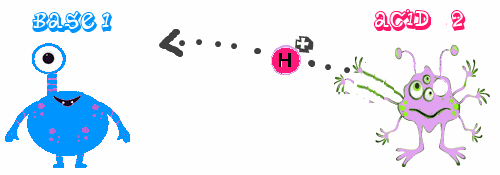Search:

# Acids and bases

## Brønstedt definitionJohannes Nikolas Brønstedt (1879-1947)

## Definitions

An acid is species which gives protons A base is a species which takes protons

## Illustration

A proton goes from an acid1 to a base2.A new acide2 is produced which holds the proton being able to give it back to the species which has been transformed to a new base1:## Vocabulary

The switchover of a proton of a Broenstedt acid to a Broenstedt base is called protolysis

## Examples

(In the following examples the acidic or basic "natures" are shown by the red or blue colour which is taken by bromothymol in acidic or basic medium)

### 1

Fluorhydric acid reacts with water:

$HF$ + $H_2O$ $\leftrightarrows$ $F^-$ + $H_3O^+$

acid 1 + base 2 $\leftrightarrows$ base 1 + acid 2

We notice that - water ( here a Brønstedt base!) receives a proton from fluorhydric acid and is transformed to the corresponding acid: the hydronium ion $H_3O^+$. - the hydrogen ion $H^+$ (= the transmitted proton $p^+$ !) is not free !

### 2

Introduced into water, ammonium chloride has an acidic nature due to the ammonium ion which reacts with water:

$NH_4^+$ + $H_2O$ $\leftrightarrows$ $NH_3$ + $H_3O^+$

acid 1 + base 2 $\leftrightarrows$ base 1 + acid 2

We notice that - the ammonium ion( a Brønstedt acid!) is not a substance ( a neutral species ). - the hydronium ion $H_3O^+$ which is present in all acidic solutions determines the acidic nature and not $H^+$ which is merely transmitted!

### 3

Introduced into water, sodium dihydrogenphosphate has an acidic nature due to due to the dihydrogenphosphate ion which reacts with water:

$H_2PO_4^-$ + $H_2O$ $\leftrightarrows$ $HPO_4^{2-}$ + $H_3O^+$

acid 1 + base 2 $\leftrightarrows$ base 1 + acid 2

We notice that a Brønstedt acid may be a molecule, a cation or even an anion ( as in this case ).

### 4

Introduced into water, ammonia has a basic nature. It reacts with water:

$NH_3$ + $H_2O$ $\leftrightarrows$ $NH_4^+$ + $OH^-$

base 1 + acid 2 $\leftrightarrows$ acid 1 + base 2

We notice that - water (here a Brønstedt acid !) gives a proton to ammonia and is transformed to the correspondingbase: the hydroxide ion $OH^-$. - water may be a Brønstedt acid or base depending on which it reacts with!

### 5

Introduced into water, sodium oxide has a basic nature due to the oxide ion which reacts with water:

$O^{2-}$ + $H_2O$ $\rightarrow$ $OH^-$ + $OH^-$

base 1 + acid 2 $\rightarrow$ acid 1 + base 2

We notice that - even the hydroxide ion may be (theorically) considered as a the Brønstedt "acid" corresponding to the oxide ion ( Brønstedt base)! - in this case the opposite reaction is practically nonexistent. That's why we write the simple dash ($\rightarrow$). That will be discussed later.

### 6Ammonia (g) reacts with hydrogen chloride (g) :

$NH_{3\;(g)}$ + $HCl_{\;(g)}$ $\rightarrow$ $NH_4^+$   $Cl^-$$_{\;(s)}$

base 1 + acid 2 $\rightarrow$ acid 1 + base 2

We notice that - the proton exchange is made without the water as a solvent! - the white smoke is ammonium chloride (solid!)

## Results

Reaction of acid and base: Acid 1 + base 2 $\leftrightarrows$ base 1 + acid 2 A special case: Acidic hydrolysis: Acid +$H_2O$ $\leftrightarrows$ base + $H_3O^+$ A special case: Basic hydrolysis Base +$H_2O$ $\leftrightarrows$ acid + $OH^-$

Acid and corresponding base make an acid - base couple for instance $(HF$,$F^-)$ $(H_2PO_4^-$,$HPO_4^{2-})$ $(NH_4^+$,$NH_3)$ $(OH^-$,$O^{2-})$...